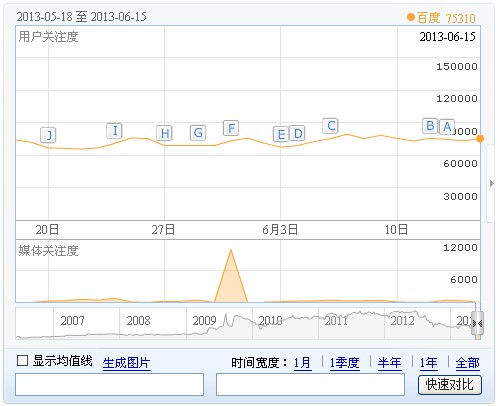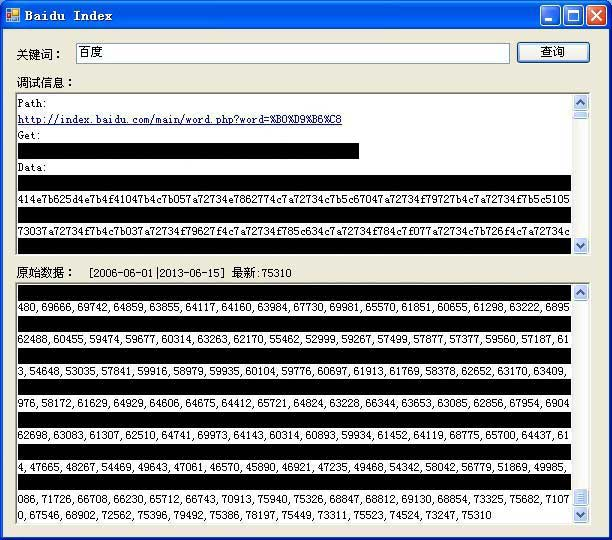﻿百度指数数据flash生成过程分析及flash加密数据采集方案研究

# 百度指数数据flash生成过程分析及flash加密数据采集方案研究

#### 百度指数，数据采集，flash解密```baidu.swf.create(
{
'id': "trendAnalyser",
'width': "492",
'height': "396",
'ver': "9.0.0",
'url': "../fla/TrendAnalyser8f7a43e0.swf",
'bgColor': "#FFFFFF",
'wmode': "transparent",
'vars':
{
keys: "百度",
areas: "0",
periods: ["2013-05-15|2013-06-13"],
periodNames: ["null"],
dv: 1,
nc: 0,
gateway: "http://index.baidu.com/gateway.php",
snapshot: "http://index.baidu.com/main/show.php",
key:"2490849166481b3801"
}
},"flashContent");
```

```            var _loc_1:String = "628136f59e0527";
var _loc_2:* = _loc_1;
_loc_2 = _loc_2;
var _loc_3:* = _loc_2.split("");
_loc_3.reverse();
_loc_2 = _loc_3.join("");
var _loc_4:* = _loc_2.split("");
_loc_2.split("").splice(Math.floor(0.805243 * 14), Math.floor(0.805243 * 14));
var _loc_5:* = "abcdefghijklmnopqrstuvwxyzABCDEFGHIJKLMNOPQRSTUVWXYZ0123456789".split("");
while (_loc_4.length < 14)
{
_loc_4.splice(Math.floor(0.805243 * _loc_4.length), 0, _loc_5[Math.floor(0.805243 * _loc_5.length)]);
}
_loc_2 = _loc_4.join("");
var _loc_6:* = _loc_2.split("");
_loc_6 = _loc_2.split("").splice(7, 14).concat(_loc_6);
_loc_2 = _loc_6.join("");
var _loc_7:* = _loc_2.split("");
var _loc_8:int = 0;
while (_loc_8 < 14)
{
_loc_7[_loc_8] = Boolean(0.805243) ? (_loc_7[_loc_8].toUpperCase()) : (_loc_7[_loc_8].toLowerCase());
_loc_8++;
}
_loc_2 = _loc_7.join("");
MovieClip.prototype.k4f1b3 = _loc_2;
```（注：本文为 [风影网络工作室] 原创文章，未经书面许可，严禁转载和复制本站的任何信息，违者必究）